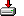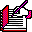#reorder.sas```%macro reorder (ds, out=);

/*
* Allow a user to reorder the variables in a dataset by writing a program that will
* rebuild the dataset.  The user moves lines within a generated attribute statement
* to change the order of the columns in the output dataset.
*
* 940512 Richard A. DeVenezia
* 001112 RAD, Rewrite to obtain meta information from DICTIONARY. only
*             instead of relying on Proc CONTENTS.  Also use a
*             SAS catalog entry to store program instead of
*             an operating system file.
*             More robust error checking.
*/

%local notes mprint symgen;
%let notes  = %sysfunc (getoption(NOTES));
%let mprint = %sysfunc (getoption(MPRINT));
%let symgen = %sysfunc (getoption(SYMBOLGEN));

options nonotes nomprint nosymbolgen;

%local ds out random reordpgm cntntout nindex lib mem;
%local mi_data;   %* will contain name of view to meta information about the data set;
%local mi_attr;   %* will contain name of view to meta information about the data set columns;
%local mi_indx;   %* will contain name of view to meta information about the data set indices;

%let ds = %upcase (&ds);
%let out= %upcase (&out);

%let random = -;

%if (%superq (ds) eq ) %then %do;
%put NOTE: No dataset was specified.;
%goto ByeBye;
%end;

%if (not %sysfunc(exist(&ds,data))) %then %do;
%put NOTE: &ds is not a table.;
%if (%sysfunc(exist(&ds,view))) %then
%put NOTE: This macro can not reorder columns of a view.;
%goto ByeBye;
%end;

%let random = %substr(%sysfunc (ranuni (0), 9.7), 3);

%let mi_data = D&random;
%let mi_attr = A&random;
%let mi_indx = X&random;

%let reordpgm = _&random;
%let nindex = 0;

filename &reordpgm catalog "WORK._REORD_._&random..source" desc="SAS Program to reorder &DS";

%*
* Determine if ds is a one-level or two-level data set name.
* A two-level name will have a period separating libname and dataset,
* a one-level name is assumed to be in libname WORK
*;

%if %index (&ds,.) %then %do;
%let lib=%scan(&ds,1,.);
%let mem=%scan(&ds,2,.);
%end;
%else %do;
%let lib=WORK;
%let mem=&ds;
%end;

%*
* If an output dataset is not specified the data step written will
* replace the input dataset
*;

%if (&out =) %then %let out = &ds;

%*
* Create view to deliver variable names, formatting and labels of input data set
* Create view to deliver index information
*;

proc sql;
create view &mi_data as select * from dictionary.tables
where libname = "&lib" and memname = "&mem" and memtype = "DATA";

create view &mi_attr as select * from dictionary.columns
where libname = "&lib" and memname = "&mem"
order by varnum;

create view &mi_indx as select * from dictionary.indexes
where libname = "&lib" and memname = "&mem"
order by idxusage, indxname, indxpos ;
quit;

%*
* Write the program that enumerates all the indices and columns
* (using an attribute statement)
*;

data _null_;

file &reordpgm;

put "data &out (";

%*
* label the output dataset same as the input dataset
*;

set &mi_data end=eomiData;
if memlabel ne '' then do;
length label \$200;
label = quote (trim(memlabel));
put @3 label=;
end;

%*
* if the dataset has any indices defined, they will be recreated using
* the code generated here
*;

n = 0;
do while (not eomiIndx);
set &mi_indx end=eomiIndx ;
by idxusage indxname;

if n = 0 then do;
put @3 "index=(";
end;
n+1;

if idxusage = 'SIMPLE' then do;
put @5 name;
end;
else do;
if first.indxname then
put @5 indxname "=(" @;
put    name @;
if  last.indxname then
put    ")" ;
end;
end;
if n then put @3 ")";

put @3 ");" ;

%*
* enumerate all the columns using an attribute statement.
* later, when the generated code is retrieved back into the program editor
* the user can move lines to rearrange the columns and otherwise
* perform mass systematic changes using find and replace
*;

put @3 "attrib" ;

do while (not eomiAttr);
set &mi_attr end=eomiAttr ;

put @5 name @;

put "length=" @;
if type = 'num'
then put " " +1 @;
else put "\$" +1 @;
put length @;

if   format ne '' then put   format= @;
if informat ne '' then put informat= @;

if label ne '' then do;
label = quote (trim(label));
put label= @;
end;

put;
end;

put @3 ";" ;

%*
* the user can place any data transformation code they prefer between the
* set and run (after the generated code has been recalled to the program editor)
*;

put "  set &ds;" ;
put "run;" ;

stop;
run;

%CleanUp:

%let xdata = %sysfunc (exist (&mi_data, view));
%let xattr = %sysfunc (exist (&mi_attr, view));
%let xindx = %sysfunc (exist (&mi_indx, view));

%if (&xdata or &xattr or &xindx) %then %do;
proc datasets nolist lib=work mt=view;
delete
%if (&xdata) %then &mi_data ;
%if (&xattr) %then &mi_attr ;
%if (&xindx) %then &mi_indx ;
;
quit;
%end;

%* load the generated code into the program editor window;

dm "pgm; clear; inc &reordpgm";

%if (%sysfunc (fileref(&reordpgm)) <= 0) %then %do;
filename &reordpgm;
%end;

%ByeBye:

options &symgen &mprint &notes;

%mend reorder;```